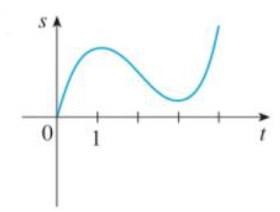Chapter 3.7, Problem 6E

Chapter
Section
Textbook Problem

Graphs of the position functions of two particles are shown, where 1 is measured in seconds. When is each particle speeding up? When is it slowing down? Explain.(a)(b)(a)

To determine

To find: When the particle is speeding up and slowing down.

Explanation

The given figure represents the position function of the particle.

It is identified from the graph that the position of the function s increases on the intervals (0, 1) and (3, 4) and s decreases on the interval (1, 3).

Velocity is positive when the position of the particle increases and the velocity is negative when the position of the particle decreases.

Moreover the acceleration is positive when the graph of the position of the particle is concave upward and it is negative when the curve is convex downward.

Thus, observe the following information.

The velocity is positive on the intervals (0, 1) and (3, 4) and the acceleration is positive on the interval (2, 4)...

(b)

To determine

To find: When the particle is speeding up and slowing down.

Still sussing out bartleby?

Check out a sample textbook solution.

See a sample solution

The Solution to Your Study Problems

Bartleby provides explanations to thousands of textbook problems written by our experts, many with advanced degrees!

Get Started

Convert the expressions in Exercises 6584 to power form. x2y

Finite Mathematics and Applied Calculus (MindTap Course List)

2 1 0 does not exist

Study Guide for Stewart's Single Variable Calculus: Early Transcendentals, 8th

True or False: If F is conservative, then curl F = 0.

Study Guide for Stewart's Multivariable Calculus, 8th